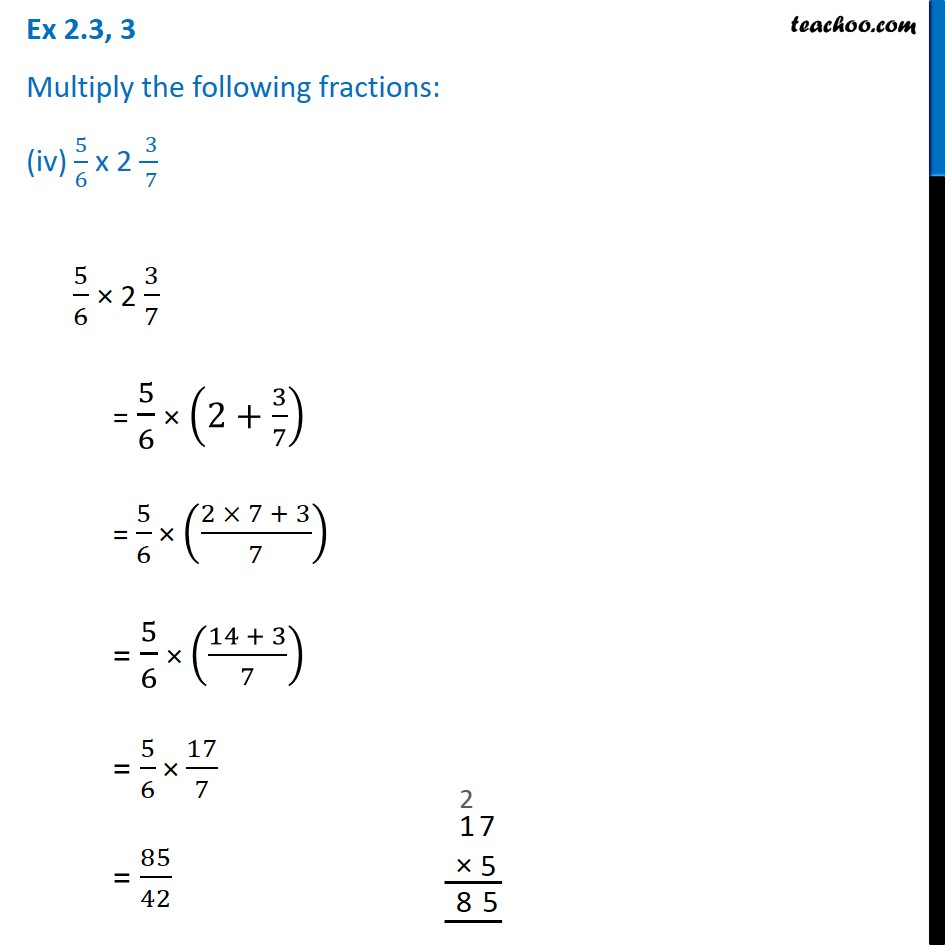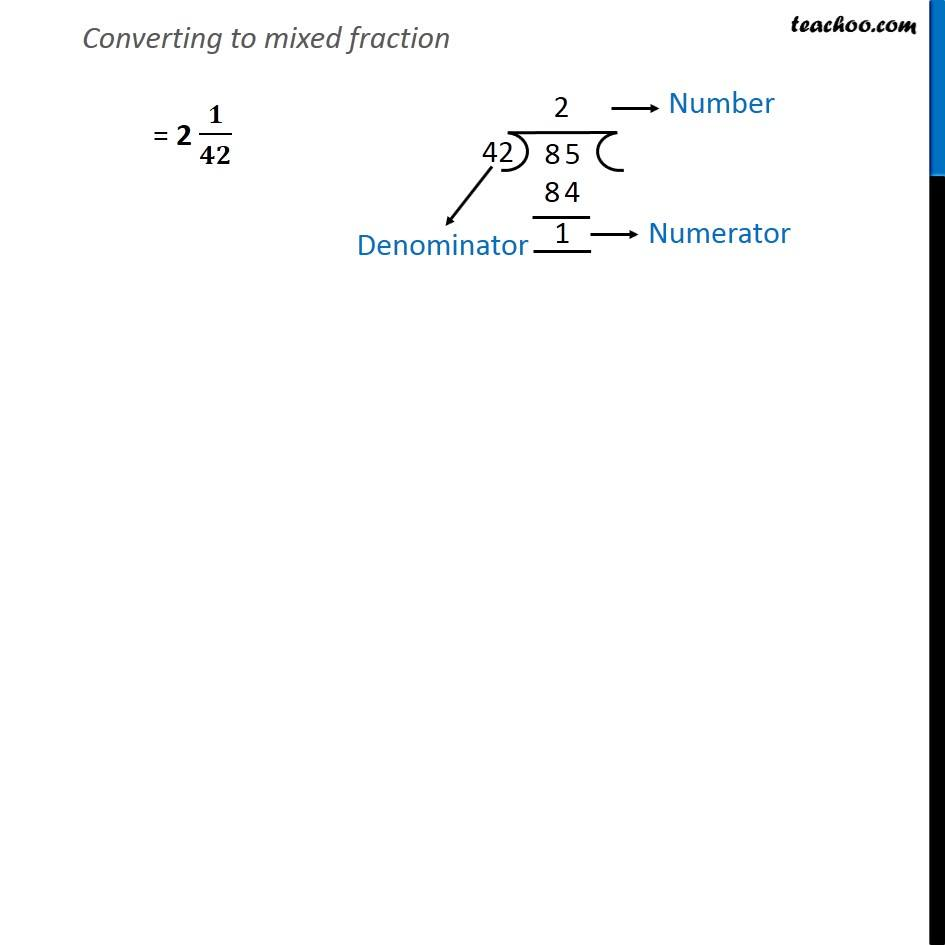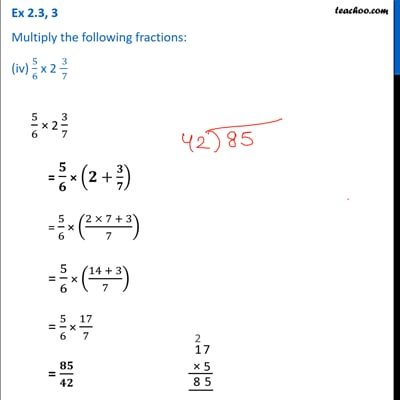Ex 2.3

Chapter 2 Class 7 Fractions and Decimals
Serial order wiseThis video is only available for Teachoo black users

### Transcript

Ex 2.3, 3 Multiply the following fractions: (iv) 5/6 x 2 ( 3)/( 7) 5/6 × 2 3/7 = 5/6 × (2+3/7) = 5/6 × ((2 × 7 + 3)/7) = 5/6 × ((14 + 3)/7) = 5/6 × 17/7 = 85/42 Converting to mixed fraction = 2 𝟏/𝟒𝟐